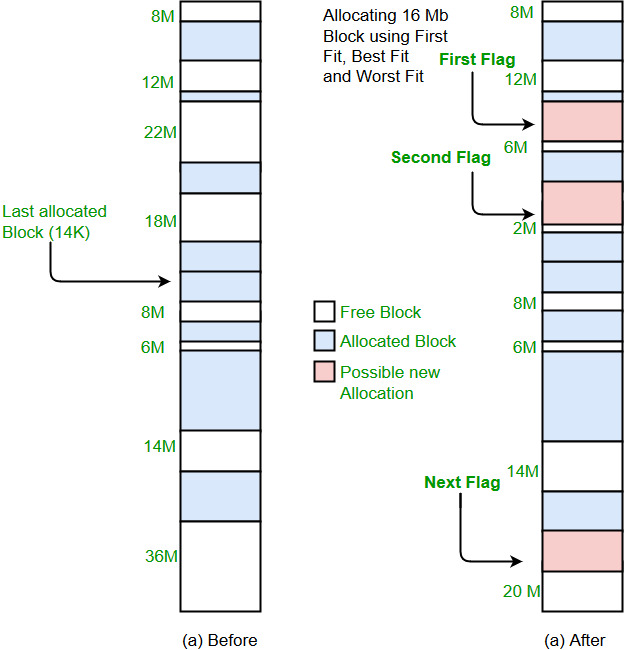Program for Worst Fit algorithm in Memory Management

• Difficulty Level : Easy
• Last Updated : 28 Jun, 2021

Prerequisite : Partition allocation methods
Worst Fit allocates a process to the partition which is largest sufficient among the freely available partitions available in the main memory. If a large process comes at a later stage, then memory will not have space to accommodate it.

Example:

Input : blockSize[]   = {100, 500, 200, 300, 600};
processSize[] = {212, 417, 112, 426};
Output:
Process No.    Process Size    Block no.
1        212        5
2        417        2
3        112        5
4        426        Not AllocatedImplementation:
1- Input memory blocks and processes with sizes.
2- Initialize all memory blocks as free.
3- Start by picking each process and find the
maximum block size that can be assigned to
current process i.e., find max(bockSize,
blockSize,.....blockSize[n]) >
processSize[current], if found then assign
it to the current process.
5- If not then leave that process and keep checking
the further processes.

Below is implementation of above steps.

C++

 // C++ implementation of worst - Fit algorithm#includeusing namespace std; // Function to allocate memory to blocks as per worst fit// algorithmvoid worstFit(int blockSize[], int m, int processSize[],                                                 int n){    // Stores block id of the block allocated to a    // process    int allocation[n];     // Initially no block is assigned to any process    memset(allocation, -1, sizeof(allocation));     // pick each process and find suitable blocks    // according to its size ad assign to it    for (int i=0; i= processSize[i])            {                if (wstIdx == -1)                    wstIdx = j;                else if (blockSize[wstIdx] < blockSize[j])                    wstIdx = j;            }        }         // If we could find a block for current process        if (wstIdx != -1)        {            // allocate block j to p[i] process            allocation[i] = wstIdx;             // Reduce available memory in this block.            blockSize[wstIdx] -= processSize[i];        }    }     cout << "\nProcess No.\tProcess Size\tBlock no.\n";    for (int i = 0; i < n; i++)    {        cout << "   " << i+1 << "\t\t" << processSize[i] << "\t\t";        if (allocation[i] != -1)            cout << allocation[i] + 1;        else            cout << "Not Allocated";        cout << endl;    }} // Driver codeint main(){    int blockSize[] = {100, 500, 200, 300, 600};    int processSize[] = {212, 417, 112, 426};    int m = sizeof(blockSize)/sizeof(blockSize);    int n = sizeof(processSize)/sizeof(processSize);     worstFit(blockSize, m, processSize, n);     return 0 ;}

Java

 // Java implementation of worst - Fit algorithm public class GFG{    // Method to allocate memory to blocks as per worst fit    // algorithm    static void worstFit(int blockSize[], int m, int processSize[],                                                     int n)    {        // Stores block id of the block allocated to a        // process        int allocation[] = new int[n];              // Initially no block is assigned to any process        for (int i = 0; i < allocation.length; i++)            allocation[i] = -1;              // pick each process and find suitable blocks        // according to its size ad assign to it        for (int i=0; i= processSize[i])                {                    if (wstIdx == -1)                        wstIdx = j;                    else if (blockSize[wstIdx] < blockSize[j])                        wstIdx = j;                }            }                  // If we could find a block for current process            if (wstIdx != -1)            {                // allocate block j to p[i] process                allocation[i] = wstIdx;                      // Reduce available memory in this block.                blockSize[wstIdx] -= processSize[i];            }        }              System.out.println("\nProcess No.\tProcess Size\tBlock no.");        for (int i = 0; i < n; i++)        {            System.out.print("   " + (i+1) + "\t\t" + processSize[i] + "\t\t");            if (allocation[i] != -1)                System.out.print(allocation[i] + 1);            else                System.out.print("Not Allocated");            System.out.println();        }    }         // Driver Method    public static void main(String[] args)    {         int blockSize[] = {100, 500, 200, 300, 600};         int processSize[] = {212, 417, 112, 426};         int m = blockSize.length;         int n = processSize.length;                   worstFit(blockSize, m, processSize, n);    }}

Python3

 # Python3 implementation of worst - Fit algorithm # Function to allocate memory to blocks as# per worst fit algorithmdef worstFit(blockSize, m, processSize, n):         # Stores block id of the block    # allocated to a process         # Initially no block is assigned    # to any process    allocation = [-1] * n         # pick each process and find suitable blocks    # according to its size ad assign to it    for i in range(n):                 # Find the best fit block for        # current process        wstIdx = -1        for j in range(m):            if blockSize[j] >= processSize[i]:                if wstIdx == -1:                    wstIdx = j                elif blockSize[wstIdx] < blockSize[j]:                    wstIdx = j         # If we could find a block for        # current process        if wstIdx != -1:                         # allocate block j to p[i] process            allocation[i] = wstIdx             # Reduce available memory in this block.            blockSize[wstIdx] -= processSize[i]     print("Process No. Process Size Block no.")    for i in range(n):        print(i + 1, "         ",              processSize[i], end = "     ")        if allocation[i] != -1:            print(allocation[i] + 1)        else:            print("Not Allocated") # Driver codeif __name__ == '__main__':    blockSize = [100, 500, 200, 300, 600]    processSize = [212, 417, 112, 426]    m = len(blockSize)    n = len(processSize)     worstFit(blockSize, m, processSize, n) # This code is contributed by PranchalK

C#

 // C# implementation of worst - Fit algorithmusing System; class GFG{    // Method to allocate memory to blocks     // as per worst fit algorithm    static void worstFit(int []blockSize, int m,                        int []processSize, int n)    {        // Stores block id of the block allocated to a        // process        int []allocation = new int[n];             // Initially no block is assigned to any process        for (int i = 0; i < allocation.Length; i++)            allocation[i] = -1;             // pick each process and find suitable blocks        // according to its size ad assign to it        for (int i = 0; i < n; i++)        {            // Find the best fit block for current process            int wstIdx = -1;            for (int j = 0; j < m; j++)            {                if (blockSize[j] >= processSize[i])                {                    if (wstIdx == -1)                        wstIdx = j;                    else if (blockSize[wstIdx] < blockSize[j])                        wstIdx = j;                }            }                 // If we could find a block for current process            if (wstIdx != -1)            {                // allocate block j to p[i] process                allocation[i] = wstIdx;                     // Reduce available memory in this block.                blockSize[wstIdx] -= processSize[i];            }        }             Console.WriteLine("\nProcess No.\tProcess Size\tBlock no.");        for (int i = 0; i < n; i++)        {            Console.Write(" " + (i+1) + "\t\t\t" + processSize[i] + "\t\t\t");            if (allocation[i] != -1)                Console.Write(allocation[i] + 1);            else                Console.Write("Not Allocated");            Console.WriteLine();        }    }         // Driver code    public static void Main(String[] args)    {        int []blockSize = {100, 500, 200, 300, 600};        int []processSize = {212, 417, 112, 426};        int m = blockSize.Length;        int n = processSize.Length;                 worstFit(blockSize, m, processSize, n);    }} // This code has been contributed by 29AjayKumar

Javascript



Output:

Process No.    Process Size    Block no.
1        212        5
2        417        2
3        112        5
4        426        Not Allocated

This article is contributed by Sahil Chhabra (akku). If you like GeeksforGeeks and would like to contribute, you can also write an article using write.geeksforgeeks.org or mail your article to review-team@geeksforgeeks.org. See your article appearing on the GeeksforGeeks main page and help other Geeks.